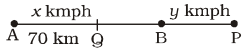## Speed, Time and Distance

#### Speed, Time and Distance

1. Points ‘A’ and ‘B’ are 70 km apart on a highway. A car starts from ‘A’ and another from ‘B’ at the same time. If they travel in the same direction, they meet in 7 hours, but if they travel towards each-other, they meet in one hour. Find the speed of the two cars (in km/hr).
1. 20, 30
2. 40, 30
3. 30, 50
4. 20, 40

1.Let speed of car starting from A be x kmph
and speed of car starting from B be y kmph
Case I
When cars meet at P,
7x = AP = AB + BP = 70 + 7y
⇒  7x – 7y = 70
⇒  x – y = 10    ...(i)
Case II
When cars meet at Q,
x + y = 70    ...(ii)
x = 40 kmph
Putting the value of x in equation (i),
y = 40 – 10 = 30 kmph

##### Correct Option: BLet speed of car starting from A be x kmph
and speed of car starting from B be y kmph
Case I
When cars meet at P,
7x = AP = AB + BP = 70 + 7y
⇒  7x – 7y = 70
⇒  x – y = 10    ...(i)
Case II
When cars meet at Q,
x + y = 70    ...(ii)
x = 40 kmph
Putting the value of x in equation (i),
y = 40 – 10 = 30 kmph

1. A train crosses a pole in 15 seconds and a platform 100 metres long in 25 seconds. Its length (in metres) is
1. 50
2. 100
3. 150
4. 200

1. Let the length of train be x metre.

 ∴ x = x + 100 15 25

 ⇒ x = x + 100 3 5

⇒  5x = 3x + 300
⇒  2x = 300
 ⇒  x = 300 = 150 metres 2

##### Correct Option: C

Let the length of train be x metre.

 ∴ x = x + 100 15 25

 ⇒ x = x + 100 3 5

⇒  5x = 3x + 300
⇒  2x = 300
 ⇒  x = 300 = 150 metres 2

1. A train passes a platform 110 m long in 40 seconds and a boy standing on the platform in 30 seconds . The length of the train is
1. 100 m
2. 110 m
3. 220 m
4. 330 m

1. Let the length of the train be x metres.

 Speed of train in crossing boy = x 30

 Speed of train in crossing platform = x + 110 40

According to the question,
 x + 110 = x 40 30

 ⇒ x + 110 = x 4 3

⇒  4x = 3x + 330
⇒  x = 330 metres

##### Correct Option: D

Let the length of the train be x metres.

 Speed of train in crossing boy = x 30

 Speed of train in crossing platform = x + 110 40

According to the question,
 x + 110 = x 40 30

 ⇒ x + 110 = x 4 3

⇒  4x = 3x + 330
⇒  x = 330 metres

1. A moving train crosses a man standing on a platform and a bridge 300 metres long in 10 seconds and 25 seconds respectively. What will be the time taken by the train to cross a platform 200 metres long ?
1.  16 2 seconds 3
2. 18 seconds
3. 20 seconds
4. 22 seconds

1. Let the length of the train be x metre

 Speed of train when it crosses man = x 10

 Speed of train when it crosses platform = x + 300 25

According to the question,
 Speed of train = x = x + 300 10 25

⇒  25x = 10x + 3000
⇒  15x = 3000
 ⇒  x = 3000 = 200 metres 15

∴  Length of train = 200 metre
 Speed of train = x = 200 10 10

 ∴ Time taken in crossing a 200 m long platform = 200 + 200 20

= 20 seconds

##### Correct Option: C

Let the length of the train be x metre

 Speed of train when it crosses man = x 10

 Speed of train when it crosses platform = x + 300 25

According to the question,
 Speed of train = x = x + 300 10 25

⇒  25x = 10x + 3000
⇒  15x = 3000
 ⇒  x = 3000 = 200 metres 15

∴  Length of train = 200 metre
 Speed of train = x = 200 10 10

 ∴ Time taken in crossing a 200 m long platform = 200 + 200 20

= 20 seconds

1. A train passes a platform 90 metre long in 30 seconds and a man standing on the platform in 15 seconds. The speed of the train is :
1. 12.4 kmph
2. 14.6 kmph
3. 18.4 kmph
4. 21.6 kmph

1. Let the length of the train be x
According to the question,

 Speed of the train = x + 90 = x 30 15

⇒  x + 90 = 2x
⇒  x = 90 m
 ∴   Speed of train = 90 15

 = 6 m/s = 6 × 18 kmph 5

= 21.6 kmph

##### Correct Option: D

Let the length of the train be x
According to the question,

 Speed of the train = x + 90 = x 30 15

⇒  x + 90 = 2x
⇒  x = 90 m
 ∴   Speed of train = 90 15

 = 6 m/s = 6 × 18 kmph 5

= 21.6 kmph